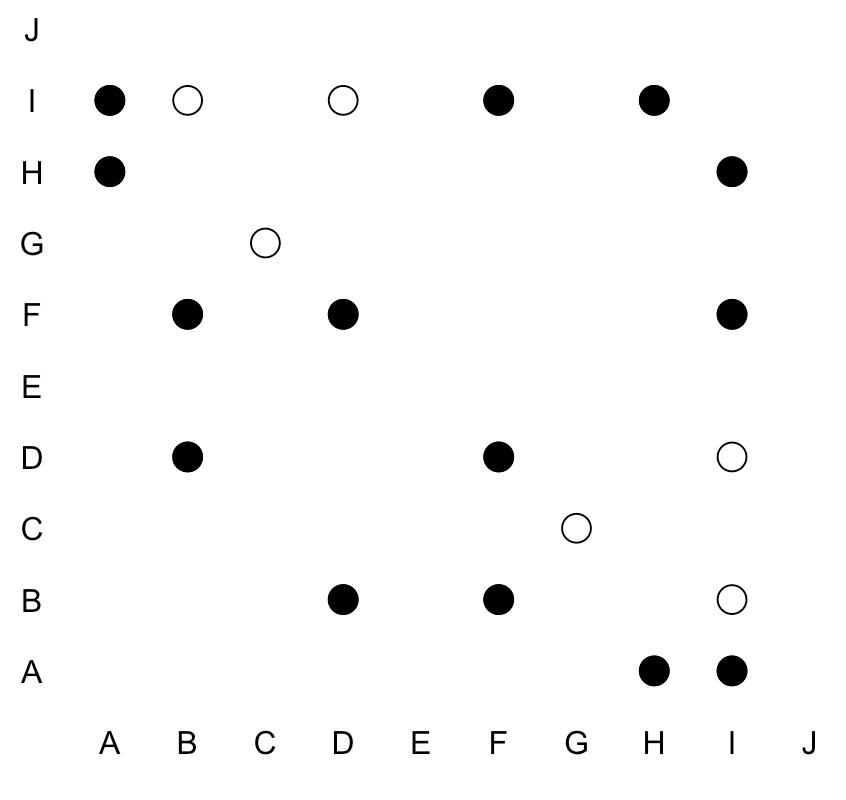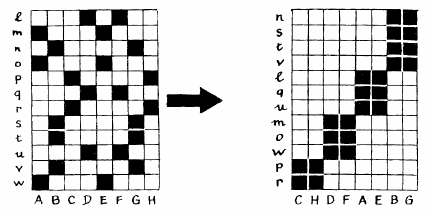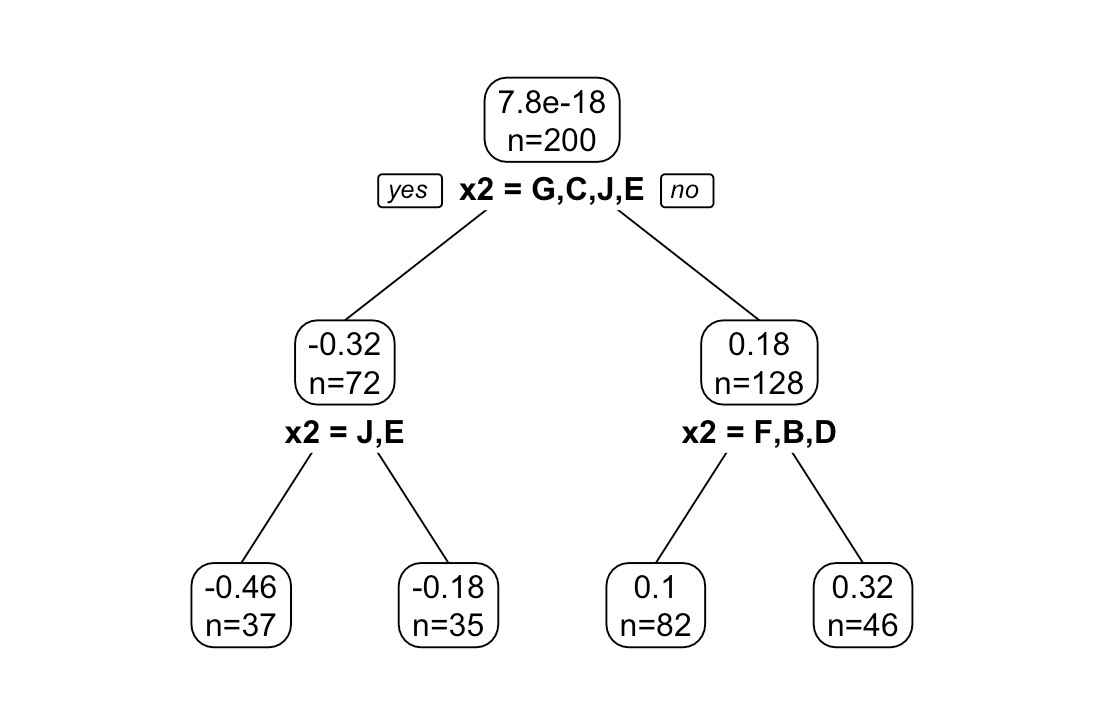# Combining automatically factor levels in R

October 6, 2018
By

(This article was first published on R-english – Freakonometrics, and kindly contributed to R-bloggers)

Each time we face real applications in an applied econometrics course, we have to deal with categorial variables. And the same question arise, from students : how can we combine automatically factor levels ? Is there a simple R function ?

I did upload a few blog posts, over the pas years. But so far, nothing satistfying. Let me write down a few lines about what could be done. And if some wants to write a nice R function, that would be awesome. To illustrate the idea, consider the following (simulated dataset)

 1 2 3 4 5 6 7 8 9 10 11 12 13 14 15 16 17 18 19  n=200 set.seed(1) x1=runif(n) x2=runif(n) y=1+2*x1-x2+rnorm(n,0,.2) LB=sample(LETTERS[1:10]) b=data.frame(y=y,x1=x1, x2=cut(x2,breaks= c(-1,.05,.1,.2,.35,.4,.55,.65,.8,.9,2), labels=LB)) str(b) 'data.frame': 200 obs. of 3 variables: $y : num 1.345 1.863 1.946 2.481 0.765 ...$ x1: num 0.266 0.372 0.573 0.908 0.202 ... $x2: Factor w/ 10 levels "I","A","H","F",..: 4 4 6 4 3 6 7 3 4 8 ... table(b$x2)[LETTERS[1:10]]   A B C D E F G H I J 11 12 23 34 23 36 12 32 3 14

There is one (continuous) dependent variable $y$, one continuous covariable $x_1$ and one categorical variable $x_2$, with here ten levels. We can plot the data using

 1 2  plot(b$x1,y,col="white",xlim=c(0,1.1)) text(b$x1,y,as.character(b$x2),cex=.5)The output of a linear regression yield the following predictions  1 2 3 4 5  for(i in 1:10){ p=function(x) predict(lm(y~x1+x2,data=b),newdata=data.frame(x1=x,x2=LETTERS[i])) u=seq(-1,1.065,by=.01) v=Vectorize(p)(u) lines(u,v)}the slope for $x_1$ is the same, we simply add a different constant for each level. As we can see, some levels are very very close, so it seems legitimate to combine them into one single category. Here is the output of the linear regression,  1 2 3 4 5 6 7 8 9 10 11 12 13 14 15 16 17 18 19 20 21 22 23 24  summary(lm(y~x1+x2,data=b)) Coefficients: Estimate Std. Error t value Pr(>|t|) (Intercept) 0.843802 0.119655 7.052 3.23e-11 *** x1 1.992878 0.053838 37.016 < 2e-16 *** x2A 0.055500 0.131173 0.423 0.6727 x2H 0.009293 0.121626 0.076 0.9392 x2F -0.177002 0.121020 -1.463 0.1452 x2B -0.218152 0.130192 -1.676 0.0955 . x2D -0.206970 0.121294 -1.706 0.0896 . x2G -0.407417 0.129999 -3.134 0.0020 ** x2C -0.526708 0.123690 -4.258 3.24e-05 *** x2J -0.664281 0.128126 -5.185 5.54e-07 *** x2E -0.816454 0.123625 -6.604 3.94e-10 *** --- Signif. codes: 0 ‘***’ 0.001 ‘**’ 0.01 ‘*’ 0.05 ‘.’ 0.1 ‘ ’ 1 Residual standard error: 0.2014 on 189 degrees of freedom Multiple R-squared: 0.8995, Adjusted R-squared: 0.8942 F-statistic: 169.1 on 10 and 189 DF, p-value: < 2.2e-16 AIC(lm(y~x1+x2,data=b))  -60.74443 BIC(lm(y~x1+x2,data=b))  -21.16463 Here the reference category is “I”. And it looks like we could actually combine that category with several others. One strategy here would be to select all categories that seem to be not significantly different, and to run a (multiple) test  1 2 3 4 5 6 7 8 9 10 11 12 13 14 15 16  library(car) linearHypothesis(lm(y~x1+x2,data=b), c("x2A = 0", "x2H = 0", "x2F = 0")) Hypothesis: x2A = 0 x2H = 0 x2F = 0 Model 1: restricted model Model 2: y ~ x1 + x2 Res.Df RSS Df Sum of Sq F Pr(>F) 1 192 8.4651 2 189 7.6654 3 0.79971 6.5726 3e-04 *** --- Signif. codes: 0 ‘***’ 0.001 ‘**’ 0.01 ‘*’ 0.05 ‘.’ 0.1 ‘ ’ 1 It seems that we can combine those four categories together. Here, we can see what’s going on when we change the reference category (actually, loop on all categories)  1 2 3 4 5 6 7 8 9 10 11 12 13 14 15 16 17  P=matrix(NA,nlevels(b$x2),nlevels(b$x2)) colnames(P)=rownames(P)=LETTERS[1:10] plot(1:nlevels(b$x2),1:nlevels(b$x2),col="white",xlab="",ylab="",axes=F,xlim=c(0,10.5), ylim=c(0,10.5)) text(1:10,0,LETTERS[1:10]) text(0,1:10,LETTERS[1:10]) for(i in 1:nlevels(b$x2)){ #levels(b$x2)=LETTERS[1:10] b$x2=relevel(b$x2,LETTERS[i]) p=summary(lm(y~x1+x2,data=b))$coefficients[-(1:2),4] names(p)=substr(names(p),3,3) P[LETTERS[i],names(p)]=p p=P[LETTERS[i],] idx=which(p>.05) points(((1:10))[idx],rep(i,length(idx)),pch=1,cex=2) idx=which(p>.1) points(((1:10))[idx],rep(i,length(idx)),pch=19,cex=2)}We are glad to see that it is symmetric : if “H” should be combined with “I”, “I” should also be combined with “H”.

Here black points are related with the 10% $p$-value, and white points the 5% $p$-value. This graph is actually hard to read… And actually, this reminds us of  Bertin (1967).Here, we can predefine manually some ordering (we will see below how it might be automatised)

 1 2 3 4 5 6 7 8 9 10 11 12 13 14 15 16 17 18 19 20 21 22  LETTERSord=c("I","A","H","F","B","D","G","C","J","E") P=matrix(NA,nlevels(b$x2),nlevels(b$x2)) colnames(P)=rownames(P)=LETTERSord plot(1:nlevels(b$x2),1:nlevels(b$x2),col="white",xlab="",ylab="",axes=F,xlim=c(0,10.5), ylim=c(0,10.5)) ct=c(3,3,2,1,1) abline(v=.5+c(0,cumsum(ct)),lty=2) abline(h=.5+c(0,cumsum(ct)),lty=2) text(1:10,0,LETTERSord) text(0,1:10,LETTERSord) for(i in 1:nlevels(b$x2)){ #levels(b$x2)=LETTERS[1:10] b$x2=relevel(b$x2,LETTERSord[i]) p=summary(lm(y~x1+x2,data=b))$coefficients[-(1:2),4] names(p)=substr(names(p),3,3) P[LETTERSord[i],names(p)]=p p=P[LETTERSord[i],] idx=which(p>.05) points(((1:10))[idx],rep(i,length(idx)),pch=1,cex=2) idx=which(p>.1) points(((1:10))[idx],rep(i,length(idx)),pch=19,cex=2) } Here we get the followingIt looks like we have our combined categories… Actually, it is possible to use another strategy. We start from some level, say “A”. Then, we merge it with all non-significantly different levels. If “B” is not one of them, we use it as the new reference. Etc.  1 2 3 4 5 6 7 8 9  for(i in 1:nlevels(b$x2)){ if(LETTERS[i]%in%levels(b$x2)){ b$x2=relevel(b$x2,LETTERS[i]) p=summary(lm(y~x1+x2,data=b))$coefficients[-(1:2),4] names(p)=substr(names(p),3,nchar(p)) idx=which(p>.05) mix=c(LETTERS[i],names(p)[idx]) b$x2=recode(b$x2, paste("c('",paste(mix,collapse = "','"),"')='",paste(mix,collapse = "+"),"'",sep="")) }}

The final categories are

 1 2 3 4  table(b$x2) A+I+H B+D+F C+G E J 46 82 35 23 14 with the following regression output  1 2 3 4 5 6 7 8 9 10 11 12 13 14 15 16 17 18 19 20  summary(lm(y~x1+x2,data=b)) Coefficients: Estimate Std. Error t value Pr(>|t|) (Intercept) 0.86407 0.03950 21.877 < 2e-16 *** x1 1.99180 0.05323 37.417 < 2e-16 *** x2B+D+F -0.21517 0.03699 -5.817 2.44e-08 *** x2C+G -0.50545 0.04528 -11.164 < 2e-16 *** x2E -0.83617 0.05128 -16.305 < 2e-16 *** x2J -0.68398 0.06131 -11.156 < 2e-16 *** --- Signif. codes: 0 ‘***’ 0.001 ‘**’ 0.01 ‘*’ 0.05 ‘.’ 0.1 ‘ ’ 1 Residual standard error: 0.2008 on 194 degrees of freedom Multiple R-squared: 0.8975, Adjusted R-squared: 0.8948 F-statistic: 339.6 on 5 and 194 DF, p-value: < 2.2e-16 AIC(lm(y~x1+x2,data=b))  -66.76939 BIC(lm(y~x1+x2,data=b))  -43.68117 Which is consistent with the group we got before. But actually, if we change the order, we can get different combinations. For instance, if we go from “J” to “A”, instead of “A” to “J”, we obtain  1 2 3 4 5 6 7 8 9 10 11 12 13 14  for(i in nlevels(b$x2):1){ #levels(b$x2)=LETTERS[1:10] if(LETTERS[i]%in%levels(b$x2)){ b$x2=relevel(b$x2,LETTERS[i]) p=summary(lm(y~x1+x2,data=b))$coefficients[-(1:2),4] names(p)=substr(names(p),3,nchar(p)) idx=which(p>.05) mix=c(LETTERS[i],names(p)[idx]) b$x2=recode(b$x2, paste("c('",paste(mix,collapse = "','"),"')='",paste(mix,collapse = "+"),"'",sep="")) }} table(b$x2)   E G+C I+A+B+D+F+H J 23 35 128 14

with different information criteria here

 1 2 3 4  AIC(lm(y~x1+x2,data=b))  -36.61665 BIC(lm(y~x1+x2,data=b))  -16.82675

I guess it would be necessary to run randomly the order we go through the levels. Last, but not least, one can use regression trees (even if it not per se in the syllabus of the course). The problem is that there is another explanatory variable that might interphere. So I would suggest (1) to fit a linear model $y=\beta_0+\beta_1x_1+u_i$, to calculate the residuals, $\widehat{u}_i$ (2) to run a regression tree, to explain $\widehat{u}_i$ with categorical variable $x_2$ (I did explain how trees are build when the explanatory variable is a categorical one in a previous post)

 1 2 3 4 5  library(rpart) library(rpart.plot) b\$e=residuals(lm(y~x1,data=b)) arbre=rpart(e~x2,data=b) prp(arbre,type=2,extra=1)Observe that the leaves have the same groups as the one we got.

 1 2 3 4 5 6 7 8 9 10 11 12 13  arbre n= 200   node), split, n, deviance, yval * denotes terminal node   1) root 200 22.563500 7.771561e-18 2) x2=G,C,J,E 72 4.441495 -3.232525e-01 4) x2=J,E 37 1.553520 -4.578492e-01 * 5) x2=G,C 35 1.509068 -1.809646e-01 * 3) x2=I,A,H,F,B,D 128 6.366628 1.818295e-01 6) x2=F,B,D 82 2.983381 1.048246e-01 * 7) x2=I,A,H 46 2.030229 3.190993e-01 *

I guess that it should be possible to put all that in an R function, to suggest combinations of level that might improve the regression.

R-bloggers.com offers daily e-mail updates about R news and tutorials on topics such as: Data science, Big Data, R jobs, visualization (ggplot2, Boxplots, maps, animation), programming (RStudio, Sweave, LaTeX, SQL, Eclipse, git, hadoop, Web Scraping) statistics (regression, PCA, time series, trading) and more...